Students can access the CBSE Sample Papers for Class 12 Chemistry with Solutions and marking scheme Term 2 Set 4 will help students in understanding the difficulty level of the exam.

## CBSE Sample Papers for Class 12 Chemistry Term 2 Set 4 with Solutions

Time Allowed: 2 Hours
Maximum Marks: 35

General Instructions:

• There are 12 questions in this question paper with internal choice.
• SECTION A – Q. No. 1 to 3 are very short answer questions carrying 2 marks each.
• SECTION B – Q. No. 4 to 11 are short answer questions carrying 3 marks each.
• SECTION C- Q. No. 12 is case based question carrying 5 marks.
• All questions are compulsory.
• Use of log tables and calculators is not allowed.

Section – A
(Section A-Question No 1 to 3 are very short answer questions carrying 2 marks each.)

Question 1.
(A) Hydrazones of aldehydes and ketones are not prepare in highly acidic medium.
Hydrazine becomes protonated in the very acidic media and hence is unable to serve as a nucleophile. As a result, extremely acidic media is not used to prepare aldehydes and ketones.

(B) Benzaldehyde gives a positive test with Tollens reagent but not with Fehlings solution. (2)
Under normal circumstances, aldehydes that lack alpha hydrogens cannot form an enolate do not produce a positive test using Fehling’s Solution, which is a weaker oxidising agent than Tollen’s reagent.

Question 2.
Rate constant k for a first order reaction has been found to be 2.54 × 10-3 sec-1. Calculate its $$\frac{3}{4}$$ th life, (log 4 = 0.6020)
OR
The rate constant for a reaction of zero order is A is 0.0030 mol L-1 s-1. How long will it take for the initial concentration of A to fall from 0.10 M to 0.075 M? (2)
For first order reaction:
t = $$\frac{2.303}{k}$$log $$\frac{a}{a-x}$$

For $$\frac{3}{4}$$th life: a – x = a – $$\frac{3}{4}$$a
On substitution these values,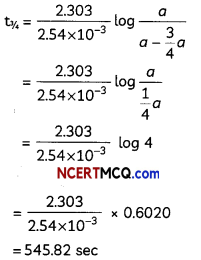OR
For a zero order reaction,
K = 0.0030 mol L-1 s-1
[A]0 = 0.10M, [A] = 0.075M
K = $$\frac{[A]_{0}-[A]}{t}$$
t = $$\frac{0.10-0.075}{0.0030}$$ = 8.33 sQuestion 3.Observe the two solutions (a) and (b), solution (b) scatters light while solution (a) does not. What do you conclude about the nature of the two solutions? (2)
When a beam of light passes through a colloid, the colloidal particles present in the solution do not allow the beam to completely pass through. The light collides with the colloidal particles and is scattered (it deviates from its normal trajectory, which is a straight line). This scattering makes the path of the light beam visible, hence Solution (b) is a colloidal solution so that path of light becomes visible while solution (a) is true solution which allows the light to pass through it.

Section – B
(Section B-Question No 4 to 11 are short answer questions carrying 3 marks each.)

Question 4.
(A) How may methyl bromide be preferentially converted to methyl isocyanide?
By carbylamine reaction:Explanation:
The carbylamine reaction is also known as Hoffmann’s isocyanide synthesis. The carbylamine reaction involves synthesis of an isocyanide when primary amine, chloroform, and a base are reacted. The reaction involves conversion of dichlorocarbene which acts to be an intermediate in the reaction.

(B) Arrange the following compounds in increasing order of solubility in water:
C6HSNH2, (C2H5)2NH, C2H5NH2
C6H5NH2 < (C2H5)2NH < C2H5NH2
Explanation:
Primary amines are less soluble than tertiary amines as primary amines can form hydrogen bonds with water but tertiary amines cannot.

(C) Why is an alkylamine more basic than ammonia? (3)
Due to electron releasing inductive effect (+1) of alkyl group, the electron density on the nitrogen atom increases and thus, it can donate the lone pair of electrons more easily than ammonia.

Question 5.
(A) On the basis of crystatfield theory, write the electronic configuration for d4 ion is ∆0 < P.
t3 2g e1g0< P, the configuration will be t2g, eg and it is in the case of weak field Ligands and high spin complex will be formed.

(B) Write the hybridization and shape of [Ni(CN)4]2-. (Atomicnumber of Ni = 28)
dsp2, square planar.

Related Theory:
The Crystal Field Stabilization Energy (CFSE) derived from the splitting of the d orbital energies is greater for square planar arrangement. In a tetrahedral arrangement, no pair of ligands is pointing at one specific d orbital. Thus, for first-row atoms such as Ni2+ (a efi species), tetrahedral arrangement will be pre-ferred if the ligands are large and weak-field. If the ligands are small (more rod-like) and are strong-field, the planar arrangement will be preferred.

(C) Which of the following is more stable complex and why?
[Co(NH3)6]3+ and [Co(en)3]3+ (3)
[Co(en)3]3+ is more stable than [Co(NH3)6]3+ as [Co(en)3]3+ is a metal chelate due to presence of bidentate ligand ethylene diamine ligand. Metal chelates are more stable than complexes containing only monodentate ligands.

Related Theory:
When a ligand attaches to the metal ion in a manner that forms a ring, then the metal- li-gand association is found to be more stable. In other words, complexes containing chelate rings are more stable than complexes without rings. This is known as the chelate effect.

Question 6.
Give reason for the following:
(A) Sky appears blue
(B) Colloidal medicines are more effective
(C) Alum is used in purfication of water
OR
Give factors governing colour of colloidal solutions. (3)
(A) Sky appears blue in colour due to scattering of light by colloidal particles. It is called Tyndall effect. It is the phenomenon in which the particles of gas or liquid get uniformly distributed throughout the body of the solid.

(B) Medicines are more effective in their colloidal state because colloids have a larger surface area. Thus, they get easily assimilated, absorbed and digested .and thus medicines in colloidal form are easily absorbed by the body tissues.

(C) Alum helps in purifying water by aluminum ion which coagulates the mud particles. Because of the high charge on the aluminum ion, it helps the small particles of suspended solids to group together until they are of such size that they sink and can be removed.
OR
The colour of the colloidal solution depends on

• the wavelength of the light scatter by the dispersed particles,
• size of the dispersed particles
• nature of the dispersed particles and the manner in which it is viewed.

Example:
Finest gold sol is red in colour and as the size of the particle keeps increasing its colour changes to blue, then purple and finally gold.Question 7.
Explain the following observations giving an appropriate reason for each:
(A) The enthalpies of atomization of transition elements are quite high.
(B) There occurs much more frequent metal- metal bonding in compounds of heavy transition metals (i.e.) 3rd series).
(C) Mn2+ is much more resistant than Fe2+ towards oxidation.
OR
Observe the table below: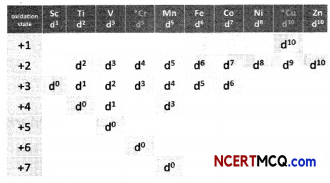(A) How is the variability in oxidation states of transition metals different from that of the non transition metals? Illustrate with examples.
(B) What may be the stabLe oxidation state of the transition element with the following d electron configurations in the ground state of their atoms: 3d3,3d53d8 and 3d4? (3)
(A) In transition elements, there are large number of unpaired electrons in their atoms, thus they have a stronger inter atomic interaction and thereby stronger bonding between the atoms. So they have high enthalpies of atomization.

(B) It is due to presence of unpaired electrons in the (n-1)d shell have tendency to make strong metallic bond by covalent nature of bonds.

(C) The 3d orbital in Mn2+ is half-filled and is more stable compared to Fe2+ having 6 electrons in the 3d orbital. Mn2+ prefer to lose an electron or get oxidised whereas Fe2+ will readily lose one electron or get oxidised. Therefore, Mn2+ is much more resistant than Fe2+ towards oxidation.
OR
(a) In case of transition elements, the variability in the oxidation state is due to participation of (n-1) d orbitals and ns orbitals.
The oxidation states differ by unity. Thus, V shows +2, +3, +4 and +5 oxidation states and Mn shows +2, +3, +4, +5, +6 and +7 oxidation states.
On the other hand, the variable oxidation states shown by some p block elements differ by two units. Thus, tin shows +2 and +4 oxidation state and indium shows +1 and +3 oxidation states.

(b) Up to Mn, the maximum stable oxidation state corresponds to sum of s and d electrons. After Mn, there is decrease in the stability of higher oxidation states. The stable oxidation states of the transition elements with the following d electron configurations in the ground state of their atoms are as shown.
3d34s2: +5
3d54s1: +1, +6
3d54s2: +2, +7
3d84s2: +2 .
3d44s2: +1, +6
The reactions can be represented at anode and at cathode in the following ways:

Question 8.
Two half-reactions of an etectrochemical cell are given below:
MnO4(aq) + 8H+(aq) + 5e → Mn2+(aq) + 4H2O
(I), E° = 1.51 V
Sn2+(aq) → Sn4+(aq) + 2e, E° = + 0.15 V.
Build a redox reaction equation from the two half-reactions and calculate the cell potential from the standard potentials also predict if the reaction is reactant or product favoured.
OR
Conductivity of 0.00250 M acetic acid is 8.00 × 10-5 cm-1. Calculate its molar conductivity. If ∆0 for acetic acid is 300.0 S cm2 mol-1 what ¡s its dissociation constant? (3)
At anode (oxidation):
[Sn2+ → Sn4+(aq) + 2e] × 5 (E° = 0.15 V)

At cathode (reduction):
MnO4(aq)(aq) + 8H+ (aq) + 5e → Mn2+(aq) + 4H2O (l)] × 2 (E° = + 1.51 V)

Cell reation:
MnO4(aq)(aq) + 16H+ + 5Sn2+ → 2Mn2+ + 5Sn4+ + 8H2O

NOW,
cell = E°cathode – E°anode
= 1.51 – 0.15 = + 1.36 V

∴ Positive value of E°cell favours formation of product.
OR
Molar ConductivityQuestion 9.
Give the structure of A, B and C in the following reactions:OR
How will you convert the following:
(A) Nitrobenzene into aniline
(B) Ethanoic acid into methanamine
(C) Aniline into N-phenylethanamide (Write the chemical equations involved) (3)OR
(A) Nitrobenzene into aniline(B) Ethanoic acid into methanomine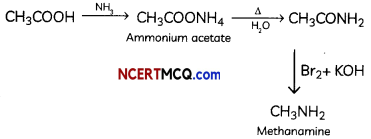(C) Aniline into N-phenytethanamideQuestion 10.
For a reaction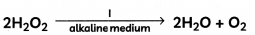The proposed mechanism is as given below:
1. H2O2 + I → H2O + IO (slow)
2. H2O2 + IO → H2O + I + O2 (fast)
(i) Write rate law for the reaction.
(ii) Write the overall order of reaction.
(iii) Out of steps (1) and (2), which one is rate-determining step? (3)
(i) To find the rate expression, slow step is to be considered as rate determining step, which is step (l) for this reaction. Then according to the rate law, Rate = k[H2O2] [I]

(ii) Since, two reagents are involved in the rate-limiting step, thus overall rate of reaction is proportional to the concentration of those reagents, that is, 2.

(iii) The slowest step in this reaction is called the rate-determining step, that is step (1).

Question 11.
(A) Give one chemical test as evidence to show that [Co(NH3)5Cl] S04 and [CO(NH3)5(S04)]Cl are ionisation isomers.
(B) [NiCl4]2- is paramagnetic while [Ni(CO)4] is diamagnetic, though both are tetrahedral. Why? (Atomic number of Ni = 28)
OR
(A) Draw figure to show the splitting of d orbitals in an octahedral crystal field.
(B) What will be the correct order for the wavelengths of absorption in the visible region for the following:
[Ni(N02)6]4-, [Ni(NH3)6]2+, [Ni(H20)6]2- (3)
(A) When [Co(NH3)5(S04)]Cl is treated with silver nitrate solution, a white precipitate of AgCl is formed. But [Co(NH3)5Cl] S04 does not give white ppt with AgN03 solution.
[Co(NH3)5S04]Cl + AgN03 → Agd (White ppt)
[CO(NH3)5 Cl]S04 + AgN03 → No (white ppt)

(B) In [NiCl4]2- complexion, nickel is in +2 oxidation state and the configuration is 3d8. Since the molecule is tetrahedral, it involves sp3 hybridisation as shown below: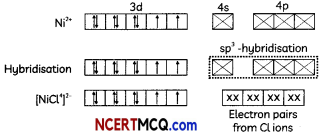The molecule is paramagnetic because it contains two unpaired electrons.
In [Ni(CO)4], nickel is in 0 oxidation state and has the configuration 4s2 3d4 or 3d10. The molecule is tetrahedral and involves sp3-hybridisation as given below: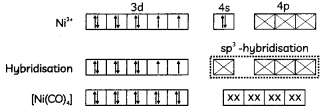Each CO donates a pair of electrons forming four Ni-CO bonds. The compound is diamagnetic since it contains no unpaired electron.
ORThe splitting of the d orbitals in an octahedral field takes place in such a way that dx2-y2, dz2 experience a rise in energy and form the eg level, while dxy dzy and dzx experience a fall in energy and form the t2g level

(B) The wavelengths of absorption in the visible region will be in the order:
[Ni(N02)6]4- > [Ni(NH3)6]2+ > [Ni(H20)6]2-

Explanation:
The central metal ion in all three complexes is the same. Therefore, absorption in the visible region depends on the ligands. The order in which the CFSE values of the ligands increases in the spectrochemical series is as follows:
H2O < NH3 < NO2-

Thus, the amount of crystal-field splitting observed will be in the following order:
0(H2O) < ∆0(NH3) < ∆0(N02 )
(More the energy, less the wavelength)Section – C
(Section C-Question No 12 is case-based question carrying 5 marks.)

Question 12.
Functional groups related to the carbonyl group include the -CHO group of an aldehyde, the -CO- group of a ketone, the -CO2H group of a carboxylic acid, and the -C02R group of an ester. The carbonyl group, a carbon-oxygen double bond, is the key structure in these classes of organic molecules: Aldehydes contain at least one hydrogen atom attached to the carbonyl carbon atom, ketones contain two carbon groups attached to the carbonyl carbon atom, carboxylic acids contain a hydroxyl group attached to the carbonyl carbon atom, and esters contain an oxygen atom attached to another carbon group connected to the carbonyl carbon atom. All of these compounds contain oxidized carbon atoms relative to the carbon atom of an alcohol group.

Aldehydes and ketones are commonly used in nature in conjunction with other functional groups. They are mainly present in microorganisms or plants and substances such as cinnamaldehyde (cinnamon bark), vanillin (vanilla bean), Citra (lemongrass), helminthosporium (a fungal toxin), carvone (spearmint and caraway), camphor (camphor trees).
For the manufacture of acetic acid and pyridine derivatives, acetaldehyde is used to a great degree.
In perfumes, beauty products, and colourants, benzaldehyde is used. It is applied and often used as a bee repellent to give almond flavour to food items.

Acetone is used in the kitchen as a nail polish remover and paint thinner.
It is used for chemical peeling and acne procedures in medicine.
(A) Name two naturally occurring aldehydes with their sources.
(B) How are ketones and aldehydes different?
(C) Write one use each of benzaldehyde and propanone.
(D) Explain the mechanism of a nucleophilic attack on the carbonyl group of an aldehyde or a ketone.
OR
Aldehydes are more reactive than ketones towards nucleophilic reagents. Explain. (5)
(A) Cinnamaldehyde (cinnamon bark), vanillin (vanilla bean),

(B) Hydrogen atom is bound to an aldehyde’s carbonyl carbon, while that of a ketone is attached to two alkyl or aryl groups. The aldehyde C-H bond makes them readily oxidisable (they are strong reducing agents).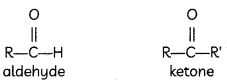(C) Benzaldehyde is used, in perfumes, beauty products, and colourants. Propanone is used as a nail polish remover and paint thinner.

(D) Mechanism of a nucleophilic attack on the carbonyl group:is a polar group in which carbon acquires positive charge and O acquires negative charge due to more electronegativity of oxygen. The Nu” attacks on carbon and forms a tetrahedral intermediate and then electrophile attacks on oxygen and forms a compound.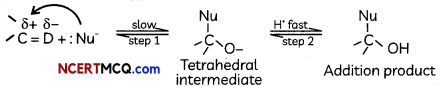OR
+I effect: Due to smaller +I effect of one alkyl group in aldehydes as compared to larger +I effect of two alkyl groups, the magnitude of positive charge on the carbonyl carbon is more in aldehydes thdn in ketones. As a result nucleophilic addition reactions occur more readily in aldehydes than in ketones.

Steric hinderance: Due to presence of a H-atom on the carbonyl group, aldehydes can be more easily oxidised than ketones. Ketones are more sterically hindered due to the presence of two bulky alkyl groups.